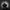# Organization of Training# Organization of Training

tags: training,

It is most important to avoid over or under training. To avoid either extreme would call for using a system to calculate the total work load for your classical lifts. You’ll be using the percentage of your one rep max and the number of lifts inside of one training day.

Let’s look at a method of periodization that has produced well over 100 all-time world records at Westside. It is referred to as the Pendulum Wave Approach. It was based on the work of Arosiev.

Westside uses a three-week wave for squatting and other speed strength training. Why three weeks and not longer? The answer is that training with an intensity of 80 percent the first week, then 85 percent and then 90 percent for five sets of five reps using Combination of Methods produced in 2019 world records for men in the squat at 148 pounds, 165 pounds and three at 123 pounds with the top of 670 pounds; plus a female all-time world record in the 132-class at 617 pounds.

Why does it work? It is a safe method to train at the correct volume no matter what your lifts are. For speed strength the bar speed should be 0.7 to 0.9 m/s. All of your sets are for speed strength; no wasted work.

How do you calculate the volume for your lifts? Simply multiply the percent—80, 85 or 90—by 25 lifts. Here’s an example: If you squat 500 pounds, your 80 percent week would be 400 pounds times 25 lifts.

500 max=80%__400x25 lifts=10,000 pounds total volume
500 max=85%__425x25 lifts=10,625 pounds total volume
500 max=90%__450x25 lifts=11,250 pounds total volume
1000 max=80%__800x25 lifts=20,000 pounds total volume
1000 max=85%__850x25 lifts=21,250 pounds total volume
1000 max=90%__900x25 lifts=22,500 pounds total volume

As you can see, 1,000 is twice the weight of 500 pounds and so the total volume is two times the volume of a 500-pound squat. This is simple math.

Westside has had 34 men squat 1,000 pounds or more and more than 100 IPF Elite Totals and those athletes have participated in experiments on all types of training including understanding intensities and volume.

Before discussing Accommodating Resistance, let’s look at one more example to calculate a 700-pound max squat.

700 max=80%__560x25 lifts=14,000 pounds total volume
700 max=80%__595x25 lifts=14,875 pounds total volume
700 max=80%__630x25 lifts=15,750 pounds total volume

As you can see, each week the volume goes up until on the fourth week you return to week one of the three-week wave and start again at 80 percent. Westside will switch to a different bar for the next three-week wave. For example, use a squat bar, a safety squat bar, bow bar or a front squat, if you like.

Now let’s turn to Accommodating Resistance. There must be a perfect weight at the bottom as well as at lockout. But, how? The answer is rubber bands.

For speed strength use approximately 33 percent band tension. This would call for a 1,000-pound squat to use 330 pounds of band tension plus 490 pounds of bar weight. An 800-pound squat would use 265 pounds of band tension and 475 pounds of bar weight. Let’s look at one more example. For a beginner who squats 300 pounds, the 80 percent of 300 pounds would use 100 pounds of band tension and 140 pounds of bar weight.

I hope this will clarify how to control your total volume at the three percents—80, 85 and 90—and no mater what you squat you will not over or under train. With close to 100 men who squat 800 pounds up to 1250 pounds, the data speaks for itself.

Stay Organized,

-Louie